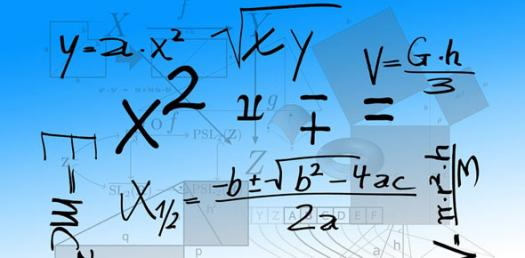# Empirical And Molecular Formula Quiz -chemistry

Approved & Edited by ProProfs Editorial Team
At ProProfs Quizzes, our dedicated in-house team of experts takes pride in their work. With a sharp eye for detail, they meticulously review each quiz. This ensures that every quiz, taken by over 100 million users, meets our standards of accuracy, clarity, and engagement.
| Written by Webdesign2012
W
Webdesign2012
Community Contributor
Quizzes Created: 1 | Total Attempts: 4,481
Questions: 10 | Attempts: 4,538SettingsDetermine the empirical and molecular formula of each compound from its percentage composition.

• 1.

### Empirical Formula: 39.8% K, 27.8% Mn, 32.5% O

• A.

N3,Mn,5O

• B.

K2,Mn,O4

• C.

Mn3,K,O7

• D.

O10,Mn,5K

B. K2,Mn,O4
Explanation
The empirical formula for the given compound is K2MnO4. This is because the percentages of each element in the compound correspond to the subscripts in the formula. The compound contains 39.8% K, 27.8% Mn, and 32.5% O, which can be approximated to a ratio of 2:1:4. Therefore, the correct formula is K2MnO4.

Rate this question:

• 2.

### Empirical Formula: 79.8% Cu, 20.2% S

• A.

Cu5,S6

• B.

S4,Cu10

• C.

Cu9,S8

• D.

Cu2,S

D. Cu2,S
Explanation
The empirical formula for the given composition of 79.8% Cu and 20.2% S is Cu2,S. This means that there are 2 atoms of copper (Cu) and 1 atom of sulfur (S) present in the empirical formula. The ratio of Cu to S in the empirical formula is 2:1, which corresponds to the given percentages. Therefore, Cu2,S is the correct empirical formula for the composition.

Rate this question:

• 3.

### Empirical Formula: 32.4% Na, 22.6% S, 45.0% O

• A.

Na4,S6,O23

• B.

S5,Na16,O

• C.

Na2,S,O4

• D.

O20,S2,Na15

C. Na2,S,O4
Explanation
The empirical formula for the given compound is Na2S0.4O. This is determined by converting the percentages of each element into moles and finding the simplest whole number ratio between them. In this case, there are 2 moles of Na, 1 mole of S, and 4 moles of O. Dividing each by the lowest number of moles (1 mole of S), we get the ratio of 2:1:4, which gives us the empirical formula Na2S0.4O.

Rate this question:

• 4.

### Empirical Formula: 62.6% Ca, 37.4% C

• A.

Ca,C2

• B.

C4,Ca10

• C.

Ca5,C3

• D.

Ca7,C10

A. Ca,C2
Explanation
The empirical formula Ca,C2 indicates that the compound contains calcium (Ca) and carbon (C) in a ratio of 1:2. This means that for every 1 atom of calcium, there are 2 atoms of carbon present. The percentages given in the empirical formula (62.6% Ca, 37.4% C) suggest that calcium is the more abundant element in the compound. Therefore, the correct answer is Ca,C2.

Rate this question:

• 5.

### Empirical Formula: 36.8%N, 63.2% O

• A.

N16,O

• B.

N2,O3

• C.

O4,N7

• D.

N2,O4

B. N2,O3
Explanation
The empirical formula of the compound with 36.8% nitrogen and 63.2% oxygen is N2O3. This is determined by converting the percentages to moles and finding the simplest whole number ratio between the elements. In this case, there are 2 moles of nitrogen for every 3 moles of oxygen, resulting in the empirical formula N2O3.

Rate this question:

• 6.

### Empirical Formula: 1.90%H, 67.6%Cl, 30.5%O

• A.

H2,O9,Cl5

• B.

Cl5,O2,H7

• C.

H,CL,O

• D.

O17,Cl3,H9

C. H,CL,O
Explanation
The empirical formula for the given compound is HClO. This is determined by converting the percentages to moles and dividing by the smallest number of moles to get the simplest ratio. In this case, the ratio is 1:1:1, which corresponds to the empirical formula HClO.

Rate this question:

• 7.

### Empirical Formula: 38.9%CL, 61.2%O

• A.

Cl2,O7

• B.

O3,Cl

• C.

O,Cl

• D.

Cl4,O8

A. Cl2,O7
Explanation
The empirical formula for the given compound with 38.9% CL and 61.2% O is Cl2O7. This is because the percentages of each element in the compound can be converted to moles, and then divided by the smallest number of moles to find the ratio of the elements. In this case, dividing the moles of Cl by 2 and the moles of O by 7 gives us the ratio of 1:3.5, which can be simplified to 1:7. Therefore, the empirical formula is Cl2O7.

Rate this question:

• 8.

### Empirical Formula: 60.0%C, 13.3%H, 26.7%O

• A.

H,C,O

• B.

H2,O4,C2

• C.

C8,O9,H3

• D.

C3,H8,O

D. C3,H8,O
Explanation
The empirical formula of the given compound is C3H8O. This can be determined by finding the simplest whole number ratio of the elements present in the compound. The percentages given indicate the relative amounts of carbon, hydrogen, and oxygen. By dividing each percentage by the atomic mass of the respective element, we can find the number of moles of each element. Then, by dividing each mole value by the smallest mole value, we can find the ratio of the elements. In this case, the ratio is 3:8:1, which corresponds to the empirical formula C3H8O.

Rate this question:

• 9.

### Molecular Formula: 65.45%C, 5.45%H, 29.10%O; mol. wt= 110

• A.

C3,H2,O5

• B.

C6,H12,O2

• C.

H3,C9,O

• D.

O4,H7,C3

B. C6,H12,O2
Explanation
The molecular formula C6H12O2 is the correct answer because it matches the given percentages of carbon, hydrogen, and oxygen. The percentages of carbon, hydrogen, and oxygen in the formula (65.45%, 5.45%, and 29.10% respectively) closely match the given percentages. Additionally, the molecular weight of the formula (110) matches the given molecular weight.

Rate this question:

• 10.

### Molecular Formula: 40.0%C, 6.7%H, 53.3%O; mol. wt= 180

• A.

456

• B.

56Back to top